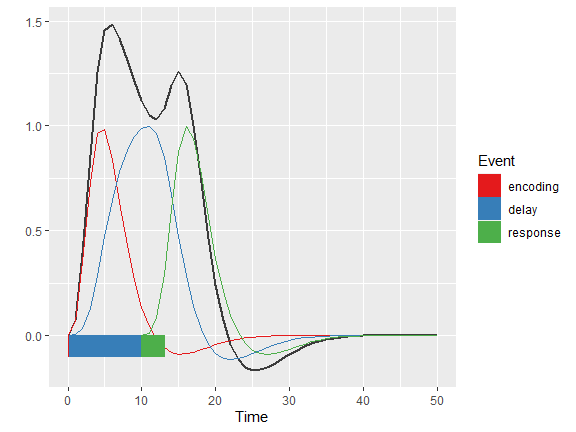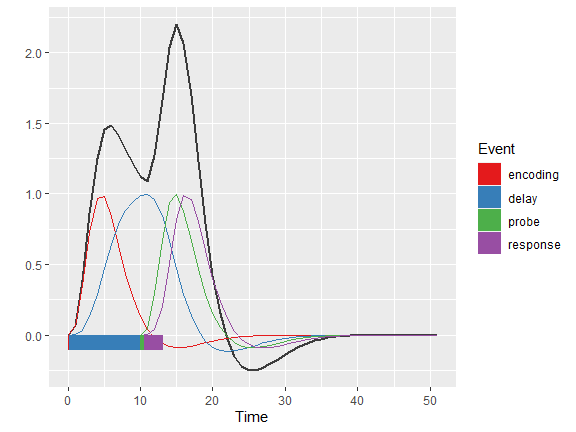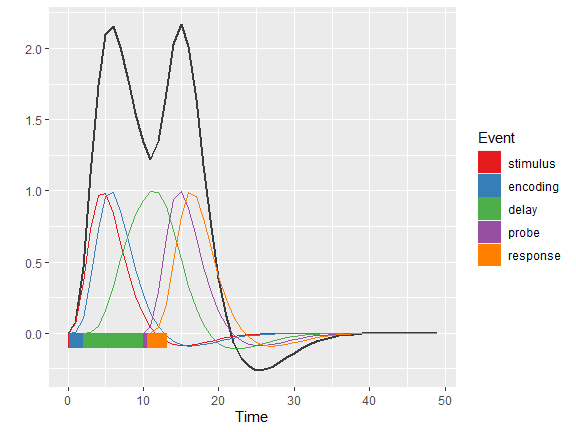# An example on how to evaluate manually constructed event models

#### 2022-11-19

The autohrf package is not only useful for preparing model specifications and then automatically finding the models that best fit the underlying data. In this example we show how you can use the autohrf package to investigate the quality of manually constructed models.

Let us start this example by loading required libraries and the data from the spatial working memory study.

# libraries
library(autohrf)

df <- swm
head(df)
##   roi t          y
## 1 L_1 0 0.02712162
## 2 L_1 1 0.06248649
## 3 L_1 2 0.12908108
## 4 L_1 3 0.30183784
## 5 L_1 4 0.51691892
## 6 L_1 5 0.65970270

The loaded data frame has 11520 observations, each with 3 variables (roi, t, and y) roi denotes the region of interest, t the time stamp and y the value of the BOLD signal. Note that input data for the autohrf package should be always organized in this manner.

Next, we construct three different models, one with three events, one with four events and one with five events. When manually constructing event models we need to create a data frame which has an entry (observation) for each of the events in the model. For each of the events we need to provide its name, its start time and its duration.

# a model with three event predictors
model1 <- data.frame(event = c("encoding", "delay", "response"),
start_time = c(0, 0.15, 10),
duration = c(0.15, 9.85, 3))

# a model with four event predictors
model2 <- data.frame(event = c("encoding", "delay", "probe", "response"),
start_time = c(0, 0.15, 10, 10.5),
duration = c(0.15, 9.85, 0.5, 2.5))

# a model with five event predictors
model3 <- data.frame(event = c("stimulus", "encoding", "delay", "probe", "response"),
start_time = c(0, 0.15, 2, 10, 10.5),
duration = c(0.15, 1.85, 8, 0.5, 2.5))

Once we construct our models we can use the evaluate_model function to obtain the model fitness to the measured data.

# evaluate models
em1 <- evaluate_model(df, model1, tr = 1)
##
## Mean R2:  0.6730635
## Median R2:  0.7901452
## Min R2:  0.008685168
## Weighted R2:  0.6730635
##
## Mean BIC:  -40.86529
## Median BIC:  -40.42351
## Min BIC:  -115.1637
## Weighted BIC:  -40.86529
em2 <- evaluate_model(df, model2, tr = 1)
##
## Mean R2:  0.8149324
## Median R2:  0.8599957
## Min R2:  0.1459216
## Weighted R2:  0.8149324
##
## Mean BIC:  -53.81372
## Median BIC:  -53.69716
## Min BIC:  -142.6516
## Weighted BIC:  -53.81372
em3 <- evaluate_model(df, model3, tr = 1)
##
## Mean R2:  0.8252129
## Median R2:  0.874879
## Min R2:  0.170891
## Weighted R2:  0.8252129
##
## Mean BIC:  -53.60671
## Median BIC:  -53.55636
## Min BIC:  -141.3314
## Weighted BIC:  -53.60671

We can also use the plot_model function to visually inspect how model fits the underlying data.

# plot models fit to the data
plot_model(em1)plot_model(em2)plot_model(em3)